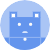search
Search
Guest 0reps
Thanks for the thanks!
close
account_circle
Profile
exit_to_app
Sign out
search
keyboard_voice
close
Searching Tips
Search for a recipe:
"Creating a table in MySQL"
Search for an API documentation: "@append"
Search for code: "!dataframe"
Apply a tag filter: "#python"
Useful Shortcuts
/ to open search panel
Esc to close search panel
to navigate between search results
d to clear all current filters
Enter to expand content previewDoc SearchCode Search BetaSORRY NOTHING FOUND!
mic
Start speaking...Voice search is only supported in Safari and Chrome.
Shrink
Navigate to
A
A
brightness_medium
share
arrow_backShareTwitterFacebook

# NumPy | reshape method

Programming
chevron_right
Python
chevron_right
NumPy
chevron_right
Documentation
schedule Jul 1, 2022
Last updated
local_offer PythonNumPy
Tags
expand_more

NumPy's `reshape(~)` method returns a new NumPy array with the desired shape. If the reshaped array contains more values than the original array, then numbers will be repeated.

# Parameters

1. `a` | `array-like`

The input array.

2. `shape` | `int` or `tuple` of `int`

The desired shape. If `-1` is passed, then the shape is inferred. Check our examples below for clarification.

# Return value

A new NumPy array with the desired shape.

# Examples

## Converting from 1D to 2D

Consider the following 1D array:

``` a = np.array([1,2,3,4,5,6])a array([1, 2, 3, 4, 5, 6]) ```

To change this to a 2D array with 2 rows and 3 columns:

``` np.reshape(a, (2,3)) array([[1, 2, 3], [4, 5, 6]]) ```

To convert a 1D array to a 2D array with one element:

``` np.reshape(a, (1, a.size)) array([[1, 2, 3, 4, 5, 6]]) ```

## Converting from 2D to 1D

Suppose we have the following 2D array:

``` a = np.array([[1,2],[3,4]])a array([[1, 2],       [3, 4]]) ```

To obtain the 1D representation:

``` np.reshape(a, 4) array([1, 2, 3, 4]) ```

Here, we could simply use the `ravel(~)` method to flatten the array:

``` a = np.array([[1,2],[3,4]])a.ravel() array([1, 2, 3, 4]) ```

## Inferring the shape

The value `-1` in `shape` tells NumPy to infer the suitable shape. For instance:

``` np.reshape([1,2,3,4], [-1,1]) array([, , , ]) ```

We could have manually specified `[4,-1]` as the shape, but the number of resulting rows can be inferred from:

• the number of values there are in the array

• the number of columns (`1` in this case).

mail